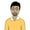# Learn React 18: Using Controlled InputsWe have now learned how to use state in React components to update information and subsequently the DOM. You will eventually have to get data from your users and then manipulate the provided information to update the component state and the DOM.

In this lesson from our free full-length beginner course on React 18, you'll learn how.

The primary way to get information from users is through forms. In React, form input elements can either be controlled or uncontrolled.

Controlled inputs are those form elements whose value is controlled by the React component state. They are the preferred way of handling form input with React. In this tutorial, we will learn the basics of controlled inputs in React by adding an input field to our random number generator from the previous tutorial.

Here is the complete code for our RandomGenerator component for reference.

 1 class RandomGenerator extends React.Component {  2  constructor(props) {  3  super(props);  4 5  this.state = {  6  randomNumber: Math.floor(Math.random() * 1000) + props.low  7  };  8  }  9 10  componentDidMount() {  11  this.numTimer = setInterval(() => {  12  let tempVal = Math.floor(Math.random() * 1000) + this.props.low;  13  this.setState({ randomNumber: tempVal });  14  }, 2000);  15  }  16 17  componentWillUnmount() {  18  clearInterval(this.numTimer);  19  }  20 21  render() {  22  return (  23 
 24 

Random Number Generator

 25 

{this.state.randomNumber}

 26 
 27  );  28  }  29 } 

Let's get started by updating the constructor method. We will add a new value to the state property to handle any changes in the range of input numbers. The initial value of numberRange comes from the value of our prop numRange. We also use the constructor to bind the handleRangeChange method to our class.

 1 constructor(props) {  2  super(props);  3 4  this.state = {  5  numberRange: props.numRange,  6  randomNumber: Math.floor(Math.random() * props.numRange) + props.low  7  };  8   9  this.handleRangeChange = this.handleRangeChange.bind(this);  10 } 

Now, we will update the code inside the render() method to include a form input. We will also update the component code to accept an additional prop for users to pass a range for generating the random numbers.

The updated render() method for the component will look like this:

 1 render() {  2  return (  3 
 4 

Random Number Generator

 5 
 6 

Lower Limit: {this.props.low}

 7 

Upper Limit: {this.props.low + this.state.numberRange}

 8 
 9 

{this.state.randomNumber}

 10 
 11   12   13 
 14  );  15 } 

As you can see, the value of our input element is set to this.state.numberRange, and we are using the onChange event to provide a function that will handle any changes in the element value. Since we are controlling the value of the input element, it is a controlled component. All the form data is being stored in the component's state.

Now we will add another method called handleRangeChange() to our component class. Here is its code:

 1 handleRangeChange(e) {  2  let newRange = parseInt(e.target.value);  3   4  if(isNaN(newRange) || newRange < 0) {  5  newRange = 0;  6  }  7   8  this.setState({numberRange: newRange});  9 } 

We get the value of the input field and make sure that it is a valid number greater than or equal to zero. Finally, we update the state to set the value of numberRange to our new input value.

The componentDidMount() method will also change to get new values based on the provided number range instead of a hard-coded limit.

 1 componentDidMount() {  2  this.numTimer = setInterval(() => {  3  let tempVal = Math.floor(Math.random() * this.state.numberRange) + this.props.low;  4  this.setState({ randomNumber: tempVal });  5  }, 2000);  6 } 

You can now render your new component on screen by using the code below:

 1 let randomElem = (  2  <>  3   4   5 );  6 7 ReactDOM.createRoot(rootElem).render(randomElem); 

Here is the CodePen demo that shows our updated RandomGenerator component with controlled input.

Try to add another input element to the component where users can also pass a lower limit for the generation of random numbers.

## Final Thoughts

When using controlled inputs, you are in charge of everything from providing a value for the input field to updating it later so that everything is handled within React. This is considered the proper way of handling form input data in React.

In the next tutorial, we will update our original random number generator to use uncontrolled input.